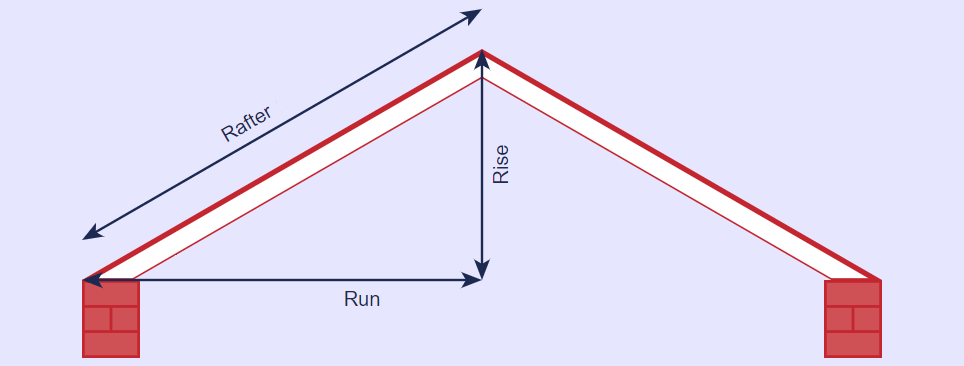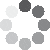(2 votes, average: 3.00 out of 5)Loading...

The roof pitch multiplier is a number that allows you to find the total surface area of your roof. Simply multiply this number by the area covered by a sloped roof to obtain the total surface area of the roof itself.  This is useful since the area of the roof itself is often difficult to measure by hand!

The formula for the roof pitch multiplier comes from the famous Pythagorean Theorem:

$$a^2+b^2 = c^2$$

Where a is the RISE of the roof, b is the RUN of the roof, and c is the RAFTER (hypotenuse) of the triangle with side lengths a and b.Now, solving for c in the equation above gives

$$c= \sqrt{a^2+b^2}$$

The roof pitch multiplier is the length of the hypotenuse divided by the RUN:

$$Roof\,pitch\,multiplier = {c \over b}$$

The chart gives a number of roof pitch multipliers for different roof slopes.

Roof Pitch Angle Roof Pitch Multiplier
1/12 4.76° 1.0035
2/12 9.46° 1.0138
3/12 14.04° 1.0308
4/12 18.43° 1.0541
5/12 22.62° 1.0833
6/12 26.57° 1.1180
7/12 30.26° 1.1577
8/12 33.69° 1.2019
9/12 36.37° 1.2500
10/12 39.81° 1.3017
11/12 42.51° 1.3566
12/12 45.00° 1.4142

Let’s see how this works in a specific example.

Contents:

## How is the roof pitch multiplier calculated?

Suppose your roof has a slope of 5/12. That is, for every 12 inches of horizontal span, the roof rises by 5 inches.  A calculator can be used to find the pitch (check out this handy calculator).

Using the Pythagorean Theorem, we find that for every 12 inches the roof spans horizontally, there are 13 inches of actual roof:

$$c = \sqrt{5^2+12^2} =\sqrt{169} = 13 \,in$$

Then the roof multiplier is

$$Roof\,pitch\,multiplier = {13 \over 12} = 1.08$$

Put it all together to arrive at a single roof pitch multiplier formula:

$$Roof\,pitch\,multiplier = {\sqrt{a^2+b^2} \over b}$$

Or, equivalently, assuming that the roof pitch is in the form RISE/RUN = x/12 gives the expression

$$Roof\,pitch\,multiplier = \sqrt{\left({rise \over run}\right)^2+1} = \sqrt{pitch^2+1}$$

## How to find the surface area of a roof

Once you know the roof slope multiplier, it is easy to calculate the surface area of the roof.  All you need to know is the area covered by the roof.

For instance, if your roof covers an area that is 20 ft by 35 ft and the pitch is 5/12, then the surface area is

$$Surface\,area = Area\,covered\,by\,roof × Roof\,pitch\,multiplier = 20 × 35 × 1.08 = 700 × 1.08 = 756\,square\,feet$$

## Step-by-step example using the roof pitch multiplier table

1. Measure the area covered by the roof. For this example, let’s assume your roof covers an area that is 20 feet wide and 40 feet long.  This means the area covered is $$Area\,covered\,by\,roof = 20 × 40 = 800\,square\,feet$$
2. Calculate the slope of the roof. You might like to use this roof slope calculator. Let’s assume the pitch is 8/12.
3. Look up the multiplier in the table, or use the Pythagorean Theorem as described above. From the table we see that a pitch of 8/12 corresponds to a multiplier of 20.
4. Multiply the area covered by the roof by the number found in Step 3 to obtain the surface area of the roof: $$Surface\,area\,of\,roof = Area\,covered\,by\,roof × Roof\,pitch\,multiplier = 800 × 1.20 = 960\,square\,feet$$(2 votes, average: 3.00 out of 5)Loading...
Related articles
Embed this calculator on your site!Add live graphics
Copied to clipboard! Preview